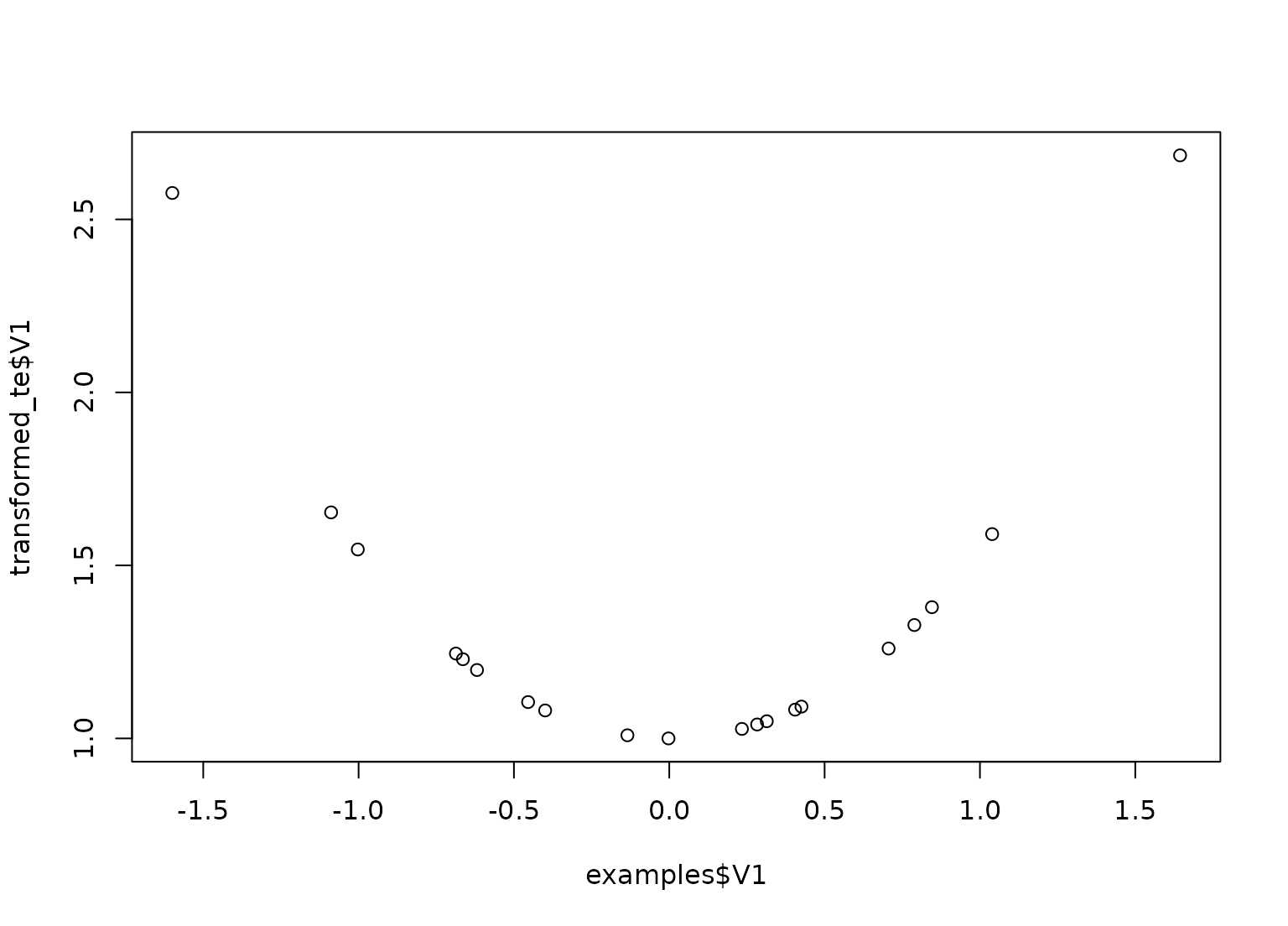step_hyperbolic creates a specification of a recipe step that will transform data using a hyperbolic function.

## Usage

step_hyperbolic(
recipe,
...,
role = NA,
trained = FALSE,
func = c("sinh", "cosh", "tanh"),
inverse = TRUE,
columns = NULL,
skip = FALSE,
id = rand_id("hyperbolic")
)

## Arguments

recipe

A recipe object. The step will be added to the sequence of operations for this recipe.

...

One or more selector functions to choose variables for this step. See selections() for more details.

role

Not used by this step since no new variables are created.

trained

A logical to indicate if the quantities for preprocessing have been estimated.

func

A character value for the function. Valid values are "sinh", "cosh", or "tanh".

inverse

A logical: should the inverse function be used?

columns

A character string of variable names that will be populated (eventually) by the terms argument.

skip

A logical. Should the step be skipped when the recipe is baked by bake()? While all operations are baked when prep() is run, some operations may not be able to be conducted on new data (e.g. processing the outcome variable(s)). Care should be taken when using skip = TRUE as it may affect the computations for subsequent operations.

id

A character string that is unique to this step to identify it.

## Value

An updated version of recipe with the new step added to the sequence of any existing operations.

## Tidying

When you tidy() this step, a tibble with columns terms (the columns that will be affected), inverse, and func is returned.

## Case weights

The underlying operation does not allow for case weights.

Other individual transformation steps: step_BoxCox(), step_YeoJohnson(), step_bs(), step_harmonic(), step_inverse(), step_invlogit(), step_logit(), step_log(), step_mutate(), step_ns(), step_percentile(), step_poly(), step_relu(), step_sqrt()

## Examples

set.seed(313)
examples <- matrix(rnorm(40), ncol = 2)
examples <- as.data.frame(examples)

rec <- recipe(~ V1 + V2, data = examples)

cos_trans <- rec %>%
step_hyperbolic(
all_numeric_predictors(),
func = "cosh", inverse = FALSE
)

cos_obj <- prep(cos_trans, training = examples)

transformed_te <- bake(cos_obj, examples)
plot(examples$V1, transformed_te$V1)tidy(cos_trans, number = 1)
#> # A tibble: 1 × 4
#>   terms                    inverse func  id
#>   <chr>                    <lgl>   <chr> <chr>
#> 1 all_numeric_predictors() FALSE   cosh  hyperbolic_IhS7o
tidy(cos_obj, number = 1)
#> # A tibble: 2 × 4
#>   terms inverse func  id
#>   <chr> <lgl>   <chr> <chr>
#> 1 V1    FALSE   cosh  hyperbolic_IhS7o
#> 2 V2    FALSE   cosh  hyperbolic_IhS7o